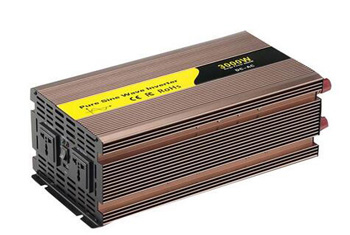# What is PWM Power Inverter?

Simply put, the power inverter is a kind of electronic device converting low-voltage (12/24/48 volts) DC into 110V/ 120V/ 220V/ 240V AC power. It is named so because we usually transform AC into DC, but the role of inverter does the opposite. The power inverter mainly adopts the PWM (Pulse Width Modulation) control technology, which can suppress or eliminate low-order harmonics. Coupled with the use of self-shutdown devices, the switching frequency is greatly increased, and the output waveform can be very close to a sine wave.

Types of power inverter

Power inverters are mainly divided into two categories, one is a sine wave inverter, and the other is a square wave inverter. Sine wave inverter output is the same as the grid we use every day or even better sine wave AC power, because it does not exist power electromagnetic pollution.Square wave inverter output is the poor quality square wave alternating current, the positive to the negative maximum value generate almost at the same time, so causing severe destabilizing effects on the load and the inverter itself.At the same time, its loading capacity is poor, only 40-60% of the rated load and can't connect inductive load (detail explained below). As the load is too large, third harmonic components of square-wave current will increase the capacitive current which flow into load.It will damage the load power supply filter capacitor when serious. For the above disadvantages, in recent years there has been quasi-sine wave (or modified sine, modified sine wave, analog sine-wave, etc.) inverter, the output waveform between the positive maximum and the negative maximum value has a time interval, effect has improved.

PWM technology in power inverter

Basic square wave inverter circuit is simple, but the output voltage waveform harmonic content is too large, and also both the THD (current THD) is too large; phase shifting small superimposed multiple harmonic content inverter output voltage waveforms. That THD is small, but the circuit is more complex. The PWM power inverter, both computer circuits, and can make the output voltage waveform, which has been widely used.The so-called PWM technology (Pulse Width Modulation, PWM) is made of a reference wave (usually a sine wave, trapezoidal wave or sometimes injected zero sequence harmonic sine wave or square wave) for modulation wave, and to N times the frequency of the modulation wave of the triangular wave (sawtooth wave is also sometimes used) is the carrier (carrier wave) comparing the waveform, the modulation wave is greater than the carrier section generates a set of equal amplitude modulated wave is proportional to the width rectangular wave pulse sequence is used to modulate the equivalent substituted for analog switch, and through the inverter switch on/off control, the direct current into alternating current, this technique is called pulse width control inverter technology. Due to carrier triangular wave (or sawtooth) dimension is a linear change of up and down, so this technique is called pulse width control inverter technology. Since the triangular carrier wave (or sawtooth) vertical width is linear, so this modulation is linear, when the modulation wave is a sine wave, pulse width of the output rectangular pulse sequence varies by sine law, this modulation technique is often another called sinusoidal pulse width modulation (Sinusoida PWM) technology.

The PWM conversion circuit has the following characteristics:

• Output voltage that is quite close to the sine wave can be obtained.
• The rectifier circuit USES diodes, which can obtain a power factor close to 1.
• Simple circuit structure.
• The output voltage can be changed by controlling the output pulse width, which speeds up the dynamic response of the frequency conversion process.

*